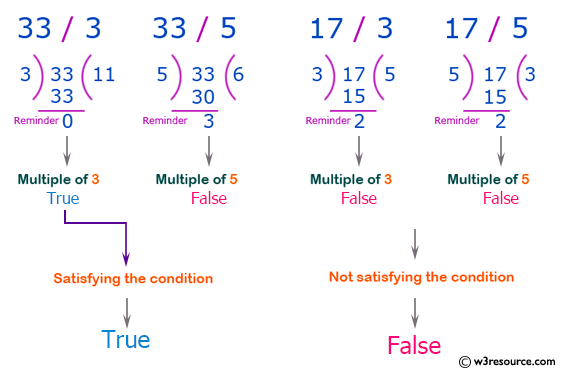﻿ Swift Basic Programming Exercise: Check if a given non-negative number is a multiple of 3 or a multiple of 5 - w3resource# Swift Basic Programming Exercise: Check if a given non-negative number is a multiple of 3 or a multiple of 5

## Swift Basic Programming: Exercise-9 with Solution

Write a Swift program to check if a given non-negative number is a multiple of 3 or a multiple of 5.

Pictorial Presentation:Sample Solution:

Swift Code:

``````func test35(num: Int) -> Bool {
if num % 3 == 0 || num % 5 == 0 {
return true
} else {
return false
}
}

print(test35(num: 33))
print(test35(num: 100))
print(test35(num: 15))
print(test35(num: 17))
```
```

Sample Output:

```true
true
true
false
```

Swift Programming Code Editor:

Improve this sample solution and post your code through Disqus

What is the difficulty level of this exercise?

﻿